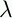September 22, 1999 Perl and the Lambda Calculus Slide #13

# Values

• If one-expression reduces to another, we say they are equivalent

• Some-expressions are irreducible (x.(x y) for example)

• If expression E is equivalent to irreducible F, F is a normal form for E

• There might be several ways to reduce an expression:

• (x.p x)((y.b) q)

• (p ((y.b) q))

• (p b)

• Or

• (x.p x)((y.b) q)

• (x.p x) b

• (p b)

 NextCopyright © 1999 M-J. Dominus